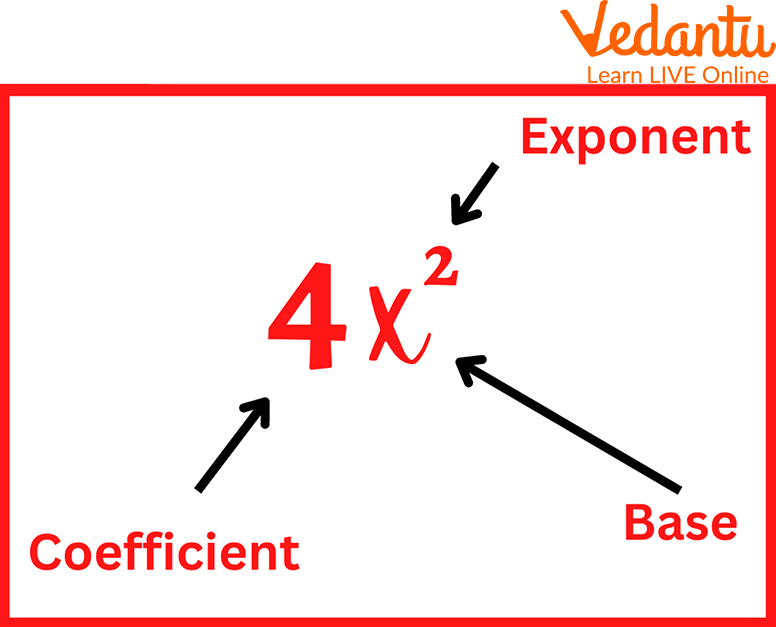Courses
Courses for Kids
Free study material
Offline Centres
More

# Addition and Subtraction of PowersLast updated date: 30th Nov 2023
Total views: 113.4k
Views today: 3.13k## Introduction of Addition of Powers

Algebra is one of the very important parts of mathematics. To understand algebra, one must know how to use exponents and radicals. The power over the given base number is known as exponents/index. For example, $4^2=4 \times 4$, where 4 is the base and 2 is the exponent. The Addition of powers is the process of adding exponents or powers of a number irrespective of whether the base is the same. In this article, we will learn about the addition and subtraction of powers with numerical bases.Introduction to Powers

## Addition and Subtraction of Powers with Numerical Bases

Addition and subtraction are the two primary operations of mathematics. But we cannot directly add or subtract powers, we can only perform addition or subtraction only on the variables that have the same base and the same power. We can only add powers in multiplication and subtract powers in the division.

$3^4+2^5=$

$=3^4$

$=3 \times 3 \times 3 \times 3$

$=81$

Also, $2^5$

$=2 \times 2 \times 2 \times 2 \times 2$

$=32$

Therefore, $3^4+2^5= 81 + 32$

$=113$

## Addition of Numbers with Powers

Adding numbers with power can be done when the base and powers are the same. There would be times when the base and powers are different, but we can still add those expressions. Let us have a look at the ways of adding powers.

Check if they got the same base and also the same power.

The usual form of addition of powers with same base is $x^{n}+x^{n}=2x^{n}$.

For example, $4^{2}+4^{2}$

$=2(4^{2})=2 \times 4 \times 4$

$=32$

If the base and power differ, then the expression will be calculated with individual terms.

The usual form is like $z^{n}+x^{m}$.

Let’s see the example for the addition of indices with different powers, $3^2+2^3=(3\times 3)(2 \times 2\times 2)=9\times8=72$.

## Addition and Subtraction of Powers

To add and subtract powers, you must first ensure that the base and power of the two terms we use to add or subtract are the same. If they are the same, then you only have to add together their coefficients and let the base and power remain identical.

$3^3+2^5 =?$

$=(3 \times 3 \times 3 )(2 \times 2 \times 2 \times 2 \times 2)$

$=(27)+(32)$

$=59$

$3^3+2^5=59$

## Laws of Indices

The First Law: Multiplication

If the two terms have identical bases (in this case, " $\mathrm{x}$ ") and are to be multiplied, their indices will be added.

Let’s see the addition of indices with different powers, $x^m \times x^n=x^{m+n}$

Example: $5^2 \times 5^1=5^{2+1}=5^3$

The Second Law is: Division

If the two terms have an identical base (in this case, " $\mathrm{x}$ ") and are to be divided, their indices will be subtracted.

$\dfrac{x^m}{x^n}=x^{m-n}$

Example: $\dfrac{2^3}{2^2}=2^{3-2} =2^{1} = 2$

The third law: Brackets

If a term with an exponent is raised to a power, then the powers are multiplied.

$\left(x^m\right)^n=x^{m \times n}$

Example: $\left(3^2\right)^2=3^{2 \times 2}=3^{4}$

## Solved Examples

Q 1. Find the value of $9^5$.

Ans: $9^5=9 \times 9 \times 9 \times 9 \times 9= 59,049$

Q 2. Find the value of $81+2^4=?$

Ans: $2^4$

$=2 \times 2 \times 2 \times 2$

$=16$

Therefore, 81 + 16 = 97.

Q 3. Find the value of $2^6+3^5$

Ans: Since $2^6+3^5$ can be written in expanded form ad

$=(2 \times 2 \times 2 \times 2 \times 2 \times 2 )+(3 \times 3 \times 3 \times 3 \times 3)$

$=(64)+(243)$

$=307$

## Practice Questions

Q 1. $\left(2^3\right)^2=$? (Ans: $64 .$)

Q 2. Find the value of $4^{-5}$. (Ans: $=\dfrac{1}{1024}$)

Q 3. $8^2 \times 4^1=$? (Ans: 256)

## Summary

In this article, we have learned about the addition of power. Adding exponents refers to the simple addition of numbers but in the form of exponents or power. This article taught us that the variables and exponents must be the same to add or subtract with powers. The power of a number tells us how many times a number is to be used in multiplication. Powers are also known as Indices or Exponents. For example, $7^{2}$ could be called “7 to the power of 2” “7’’to the second power”, or simply “7 squared”. In the end, we added the practice problem to check the command over the topic. So after going through the article, give it a try!

## FAQs on Addition and Subtraction of Powers

1. Are exponent and power the same in maths?

The power over the given base number is known as exponents/index. For example, $4^{2}= 4 \times 4$, where 4 is the base and 2 is the exponent. So yes, they are the same.

2. What is a Negative Exponent?

The negative exponent tells us how many times we have to multiply the reciprocal of the base. For the example of negative exponents $3^{-2}$. Hence, the value of $3^{-2}$ is $\dfrac{1}{9}$

3. What is a Zero Exponent?

A number having a power zero is termed a zero exponent. Those number values are always 1. Any number with the exponent 0 equals 1.